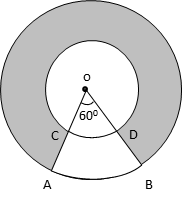# CBSE 2017 Board Solution

Class 10 Math - Set 3 - Q16. Mensuration

#### Question In the given figure, two concentric circles with centre O have radii 21 cm and 42 cm. If ∠AOB = 60°, find the area of the shaded region. (Use π = $$frac{22}{7}$) #### Video Explanation #### Explanatory AnswerRadius of inner circle = 21cm Radius of outer circle = 42cm Area of the complete disc = area of outer circle – area of inner circle = π$router)2 – π(rinner)2

But an arc AB subtending 60° is not shaded.
So, area of shaded region = $$frac{300}{360}$ × area of complete disc = $\frac{5}{6}$$π(42)2 - π(21)2)
= $$frac{5}{6}$ π$422 – 212)
= $$frac{5}{6}$ π[$42 + 21)(42 – 21)]
= $$frac{5}{6}$ π$63 × 21)
= $$frac{5}{6}$ × $\frac{22}{7}$$63 × 21)
= 55 × 63
= 3465 cm2

.